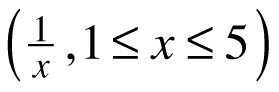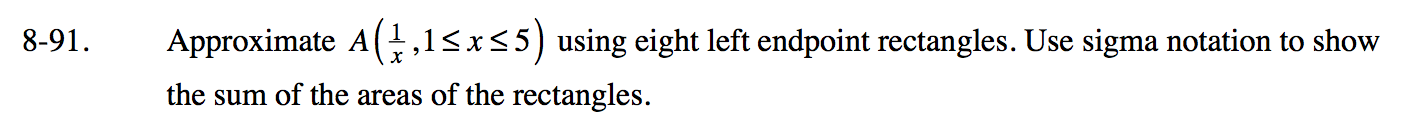### Home > PC3 > Chapter 8 > Lesson 8.3.1 > Problem8-91

8-91.

Approximate Ausing eight left endpoint rectangles. Use sigma notation to show the sum of the areas of the rectangles. Homework Help ✎Determine the width of each rectangle.

$\frac{\text{length of interval}}{\text{number of rectangles}}$

Write an equation for the xns.

x0 = 1, x1 = 1.5, ...

Use sigma notation to show the sum of the areas of the 8 rectangles.

$\text{width}\sum_{n=0}^{7}\frac{1}{\text{(expression for }x_n\text{s})}$